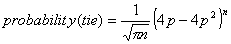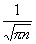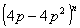Economics 849 Midterm

Prof. Bryan Caplan

Fall, 2001

Part 1: True, False, and Explain

(10 points each - 2 for the right answer, and 8 for the explanation)

State whether each of the following six propositions is true or false.  In 2-3 sentences (and clearly-labeled diagrams, when helpful), explain why.

1.  T, F, and Explain:  In the real world, coercive solutions to public goods problems may be Pareto improvements, but not Kaldor-Hicks improvements.

FALSE.  All Pareto improvements are K-H improvements, but not vice versa.  In theory, coercion can create Pareto improvements by overcoming strategic behavior (lying about your preferences, holding out for a better deal, etc.).  In the real world, though, preferences are heterogeneous enough that coercive solutions will never be Pareto improvements.  They might still be K-H improvements, though.

2. T, F, and Explain:  If you quadruple N, where (2N+1) is the number of votes, you halve the probability that one vote will be decisive.

FALSE.  This ignores the second part of the decisiveness formula that kicks in whenever a perfect 50/50 split is not expected.  Probability of the decisiveness is given by:.  If you quadruple N, then theterm does fall by half:=2*.  But quadrupling N further reducesunless p=.5.

3. T, F, and Explain:  A per-unit tax will be efficiency-enhancing iff d>1.

TRUE.  Since the slope on the demand curve is 1, d=1 indicates that demand and SB perfectly overlap.  If d>1, however, SB lies below the demand curve, indicating negative externalities.  In principle a per-unit tax can be efficiency-enhancing with negative externalities.

4.  You can derive a formula for the difference between equilibrium quantity Q and optimal quantity Q* as a function of d.

T, F, and Explain:  That formula is: Q-Q*=d/(1+d).

FALSE.  First, solve for Q by setting S=D.  P=1-P implies that P=.5, and since Q=P, Q=.5.  Second, solve for Q* by setting S=SB.  P=1-dP implies that P=1/(1+d), and since Q=P, Q=1/(1+d) as well.  Then just take the difference: Q-Q*=1/2-1/(1+d), which simplifies to Q-Q*=[d-1]/2[1+d].

An easier answer I would have accepted would simply have substituted d=1 into d/(1+d).  At d=1, there is no externality, so Q-Q* should equal 0.  But it in fact equals .5, indicating that this formula is false.

5.  Cooter explains how judges are able to "reinterpret" laws in directions they favor.

T, F, and Explain:  The only reason this works, according to Cooter, is that legislatures find reversing judicial decisions is too time-consuming.

FALSE.  What Cooter shows is that judges can reinterpret laws by making sure they move to new points that lie inside the Pareto set.  If they remain within the Pareto set, there will not be a Minimum Winning Coalition willing to overturn the judicial decision.  This would be true even if time were free.

6.  Assume that Supply is given by Q=3P, Demand by Q=10-.5P, and there are no externalities.  Government sets a per-unit tax t to maximize the SUM of (tax revenue + consumers' surplus + producers' surplus).

T, F, and Explain:  The solution to the government's optimization problem is given by t*= 30/7.

FALSE.  The solution is t*=0!  Per-unit taxes have DW costs.  Since there are no externalities, and no revenue constraint, government maximizes (revenue + CS + PS) only by refraining from imposing any per-unit tax.

The answer would still be false if government were a revenue-maximizer: then tQ(t) would reach a maximum at t*=10.

Part 2: Short Essays

(20 points each)

In 6-8 sentences, answer all of the following questions.

1.  Max Stearns of the GMU law school argues that it IS in individuals' self-interest to vote because in the real world, politicians pay attention to vote share, not just whether they won or lost.  In other words, a vote does not have to be decisive to change policy.  Is Stearns right or wrong?  Why or why not?

Stearns is wrong, though he raises an interesting point.  Politicians do sometimes seem to want a "mandate" for change, and the larger their victory margin, the stronger they say their mandate is.  But this is irrelevant to selfish voting.  In the standard model, voters have an infinitesimal chance of changing who wins.  In the Stearns model, voters are certainly able to effect an infinitesimal change.  In both cases, self-interest argues against trading a finite amount of time for an infinitesimal benefit.

2.  It frequently happens that two senators who represent  the same state disagree.  Is there any way for the Median Voter Theorem to explain this?  Explore two theoretical possibilities and assess their empirical plausibility.

Senatorial elections are staggered - 1/3 face election every two years.  Therefore, two senators from the same state may not have been elected at the same time.  One way for the MVT to handle this divergence, then, is to say that citizen preferences shifted during the interim.  A different way is to say that citizen preferences stayed the same, but voter turnout changed in a skewed way.  For example, the senator who runs during the same year as the presidential election may face a different median voter due to higher (and skewed participation).

A third possibility that no one raised is that inter-state migration might affect things.

(Students suggested a number of other possibilities, but I took off points the further the answers strayed from the standard Median Voter Theorem assumptions).

3.  Many researchers use the Principle of Aggregation to argue that voter ignorance does not have serious policy consequences.  What empirical findings from Delli Carpini and Keeter - if any - suggest the opposite?  Carefully explain your answer.

Delli Carpini and Keeter show that voter knowledge is higher in certain sub-groups in the population: the well-educated and males, to take two examples.  Given selfish voting, this could skew policy in the direction of these group's interests.  Selfish voting combined with group differences is key here, though: if the well-educated and males were voting in the public interest, the Miracle of Aggregation still holds.

Furthermore, widespread ignorance about the basic "rules of the game" - well-documented by Delli-Carpini and Keeter - raises questions about the Principle of Aggregation.  If only 10% of all voters know, say, that spending bills must originate in the House, and the other 90% are equally likely to blame the House, the Senate, or the President for fiscal mismanagement, 60% blame the wrong body.  It is unclear how aggregation solves this problem.